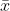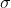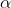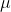In: Math

# A data set includes 103 body temperatures of healthy adult humans having a mean of 98.5°F...

A data set includes 103 body temperatures of healthy adult humans having a mean of 98.5°F and a standard deviation of 0.61°F. Construct a 99​% confidence interval estimate of the mean body temperature of all healthy humans. What does the sample suggest about the use of 98.6°F as the mean body​ temperature?

What is the confidence interval estimate of the population mean μ​?

°F < μ < °F

​(Round to three decimal places as​ needed.)

## Solutions

##### Expert Solution

Solution :

Given that,
sample mean == 98.5

Population standard deviation ==0.61

Sample size = n =103

At 99% confidence level the z is ,= 1 - 99% = 1 - 0.99 = 0.01/ 2 = 0.01 / 2 = 0.005

Z/2 = Z0.005 = 2.576

Margin of error = E = Z/2* (/n)

= 2.576* ( 0.61/ 103)

= 0.155

At 99% confidence interval estimate of the population mean is,- E <<+ E

98.5-0.155 << 98.5+0.155

°F98.345<<98.655 °F

(, )

## Related Solutions

##### A data set includes 103 body temperatures of healthy adult humans having a mean of 98.9...
A data set includes 103 body temperatures of healthy adult humans having a mean of 98.9 F and a standard deviation of 0.67 F. Construct a 99​% confidence interval estimate of the mean body temperature of all healthy humans. What does the sample suggest about the use of 98.6F as the mean body​ temperature? What is the confidence interval estimate of the population mean ​?
##### A data set includes 109 body temperatures of healthy adult humans having a mean of 98.3degrees°F...
A data set includes 109 body temperatures of healthy adult humans having a mean of 98.3degrees°F and a standard deviation of 0.54 degrees°F. Construct a 99​% confidence interval estimate of the mean body temperature of all healthy humans. What does the sample suggest about the use of 98.6 degrees°F as the mean body​ temperature?
##### A data set includes 104 body temperatures of healthy adult humans having a mean of 98.7degrees°F...
A data set includes 104 body temperatures of healthy adult humans having a mean of 98.7degrees°F and a standard deviation of 0.66degrees°F. Construct a 99​% confidence interval estimate of the mean body temperature of all healthy humans. What does the sample suggest about the use of 98.6degrees°F as the mean body​ temperature?
##### A data set includes 109109 body temperatures of healthy adult humans having a mean of 98.298.2degrees°F...
A data set includes 109109 body temperatures of healthy adult humans having a mean of 98.298.2degrees°F and a standard deviation of 0.620.62degrees°F. Construct a 9999​% confidence interval estimate of the mean body temperature of all healthy humans. What does the sample suggest about the use of 98.6degrees°F as the mean body​ temperature? What is the confidence interval estimate of the population mean muμ​?